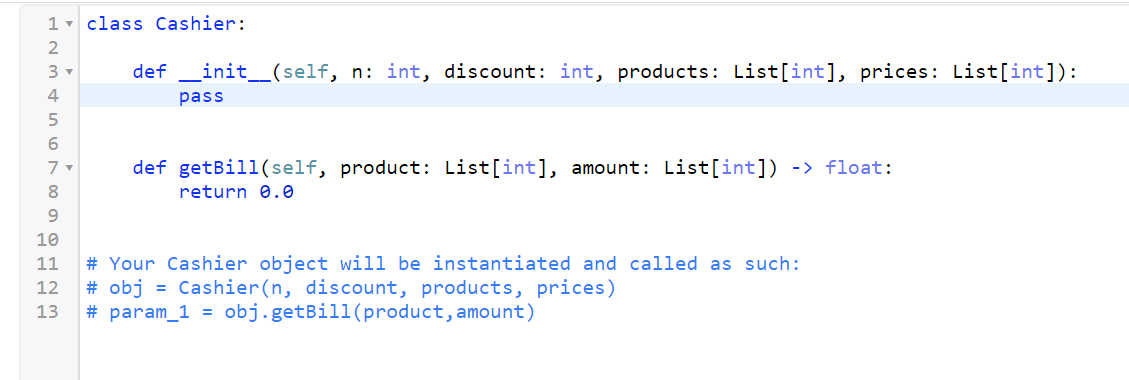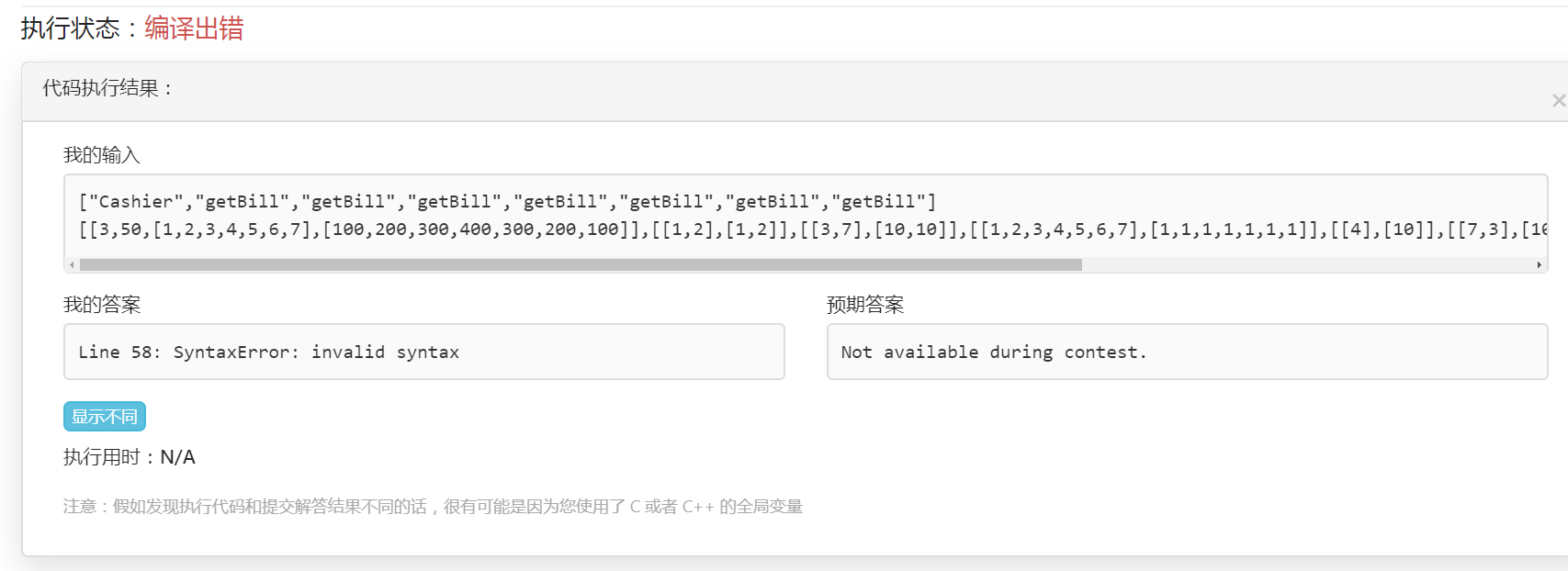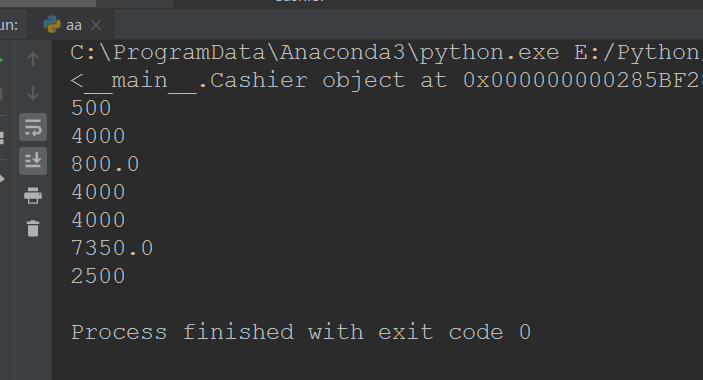🐱 第 20 场夜喵双周赛

T2的python3版本编译是不是有问题？直接提交会编译失败15

65

3

A. STL题
B. 简单模拟
C. 二分
D. 数学推理

#### Problem A

class Solution {
public:
vector<int> sortByBits(vector<int>& arr) {
sort(arr.begin(), arr.end(), [](int a, int b) {
if (__builtin_popcount(a) == __builtin_popcount(b))
return a < b;
else
return __builtin_popcount(a) < __builtin_popcount(b);
});
return arr;
}
};


#### Problem B

class Cashier {
public:
int n, d;
map <int, int> p;
int cnt;
Cashier(int _n, int discount, vector<int>& products, vector<int>& prices) {
n = _n;
d = discount;
for (int i = 0; i < products.size(); i++) p[products[i]] = prices[i];
cnt = 0;
}

double getBill(vector<int> product, vector<int> amount) {
double ans = 0;
for (int i = 0; i < product.size(); i++) ans += amount[i] * p[product[i]];
cnt++;
if (cnt == n) {
cnt = 0;
ans = ans - (d * ans) / 100;
}
return ans;
}
};


#### Problem C

class Solution {
public:
int pref;
bool check(int p, int x, int orig) {
for (int i = 0; i < 3; i++) {if (pref[i][x] - pref[i][p - 1] < 1) return false;}
return true;
}
int numberOfSubstrings(string s) {
int ans = 0;
s = "\$" + s;    //习惯从1开始，避免前缀和第一项的特判
memset(pref, 0, sizeof(pref));
int n = s.length() - 1;
for (int i = 1; i <= n; i++)
for (int j = 0; j < 3; j++) {
pref[j][i] = pref[j][i - 1] + (s[i] - 'a' == j);
}
for (int i = 1; i <= n; i++) {
int L = i + 1, R = n + 1;
while (L + 1 < R) {
int mid = (L + R) >> 1;
if (check(i, mid, s[i] - 'a')) {
R = mid;
}
else L = mid;
}
ans += n - L;
}
return ans + 1;
}
};


#### Problem D

const int MOD = 1e9 + 7;
class Solution {
typedef long long LL;
LL qpow(LL a, LL b, LL p = MOD) {
LL ans = 1;
for (; b; b >>= 1) {
if (b & 1) ans = ans * a % p;
a = a * a % p;
}
return ans;
}
LL fact(int x) {
LL ans = 1;
for (int i = 1; i <= x; i++) ans = ans * i % MOD;
return ans;
}
LL getInv(LL x) {
return qpow(x, MOD - 2);    //求逆元
}
public:
int countOrders(int n) {
return (int)((long long)(fact(2 * n) * getInv(qpow(2, n))) % MOD);
}
};

3

T1:

T2:

T3:

tail指针先找到第一个满足abc都有的序列。显然后面的所有序列都满足。 则有len + 1 - tail个。

T4:

2

1

1

1

1